# Scientific Notation Rational and Irrational Numbers Scientific Notation

• Slides: 20Scientific Notation Rational and Irrational NumbersScientific Notation 4. 632 x Coefficient is 4. 632 6 10 Base is 10 Exponent is 6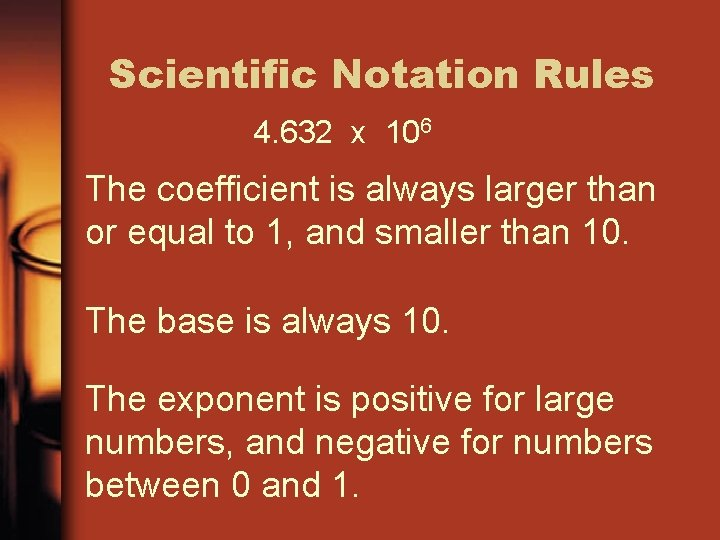Scientific Notation Rules 4. 632 x 106 The coefficient is always larger than or equal to 1, and smaller than 10. The base is always 10. The exponent is positive for large numbers, and negative for numbers between 0 and 1.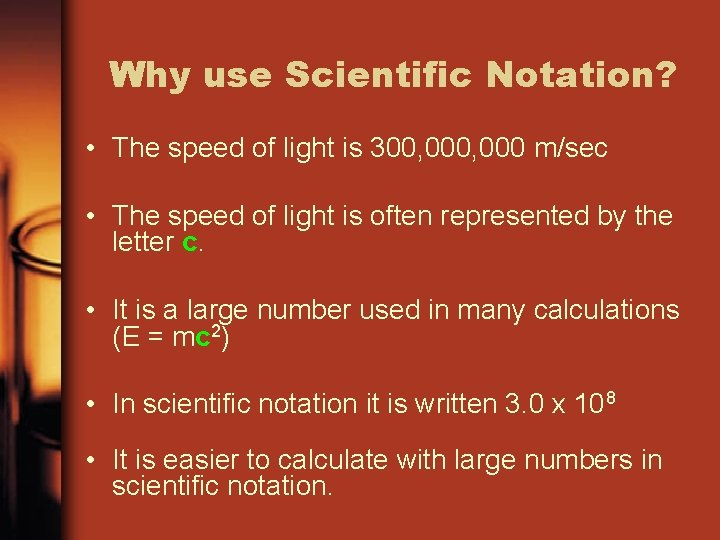Why use Scientific Notation? • The speed of light is 300, 000 m/sec • The speed of light is often represented by the letter c. • It is a large number used in many calculations (E = mc 2) • In scientific notation it is written 3. 0 x 108 • It is easier to calculate with large numbers in scientific notation.How does Scientific Notation Work? • 14 0, 000, 000 • 1. 4 x standard form What number goes here? ? 10 • 1. 4 x 1011 scientific notationScientific Notation: Great for very small numbers • The size of a dust particle is about 0. 000 000 752 kilograms • 0. 000 000 752 • 7. 52 standard form x 10? what number goes here? • 7. 52 x 10 -10 scientific notationWhat does Scientific Notation look like on the HP 39 G ? Find the EEX key To access these second functions, you need to hit the SHIFT keyUsing the HP 39 G • Enter the following number: • 3. 4 x 1011 • 3. 4 shift EEX 11 enter • Standard form: 340, 000, 000 • Notice that both the scientific form and the standard form are displayed on the screen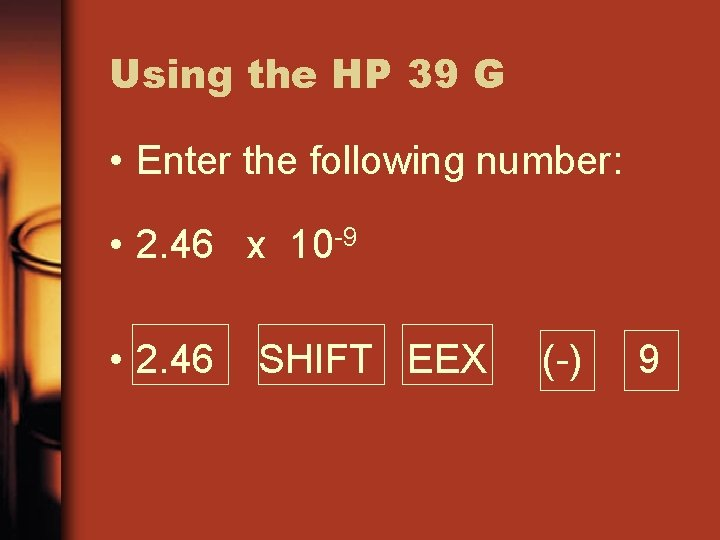Using the HP 39 G • Enter the following number: • 2. 46 x 10 -9 • 2. 46 SHIFT EEX (-) 9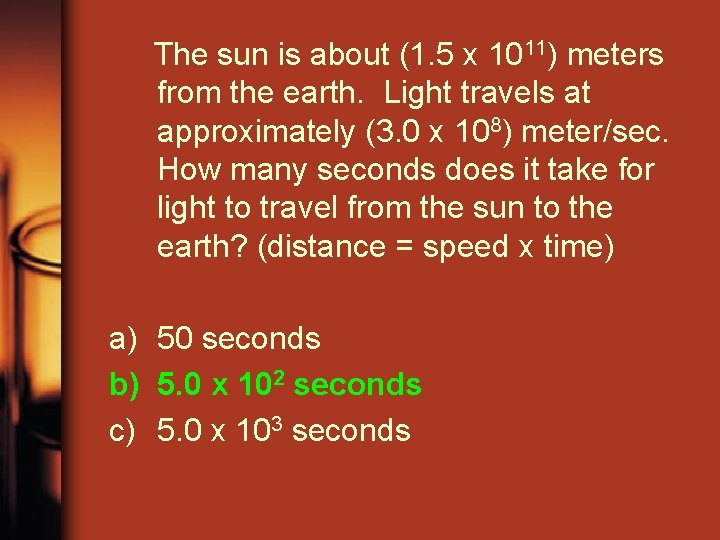The sun is about (1. 5 x 1011) meters from the earth. Light travels at approximately (3. 0 x 108) meter/sec. How many seconds does it take for light to travel from the sun to the earth? (distance = speed x time) a) 50 seconds b) 5. 0 x 102 seconds c) 5. 0 x 103 seconds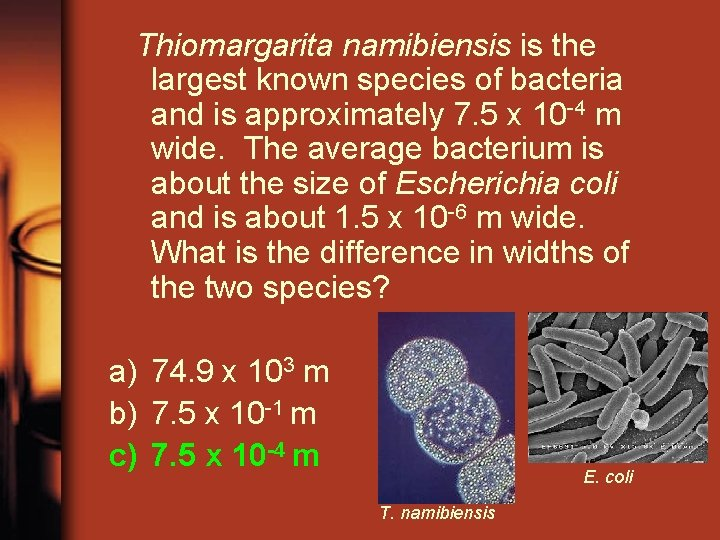Thiomargarita namibiensis is the largest known species of bacteria and is approximately 7. 5 x 10 -4 m wide. The average bacterium is about the size of Escherichia coli and is about 1. 5 x 10 -6 m wide. What is the difference in widths of the two species? a) 74. 9 x 103 m b) 7. 5 x 10 -1 m c) 7. 5 x 10 -4 m E. coli T. namibiensisSolve and express the answer in scientific notation: ( 2. 45 x 105 ) x (5 x 103 ) a) 1. 225 x 109 b) 12. 25 x 108 c) 1. 225 x 1015 d) 12. 25 x 1015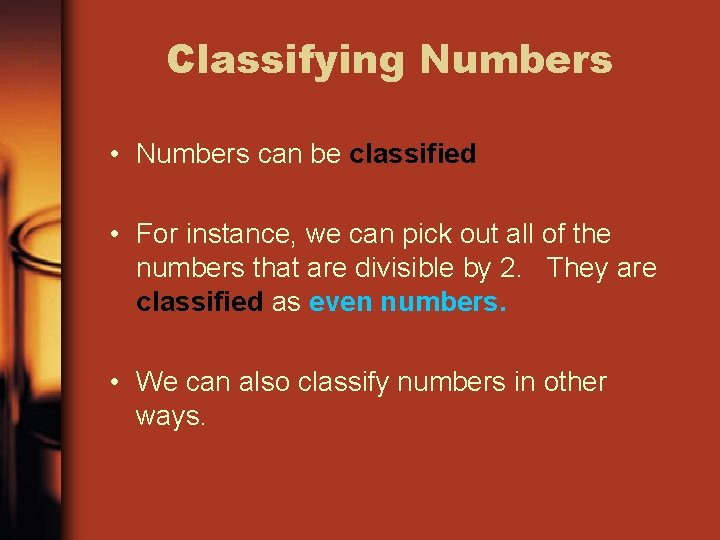Classifying Numbers • Numbers can be classified • For instance, we can pick out all of the numbers that are divisible by 2. They are classified as even numbers. • We can also classify numbers in other ways.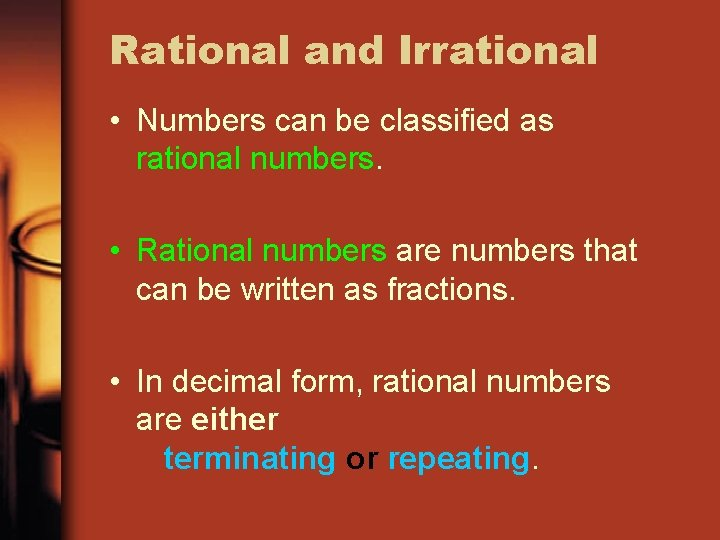Rational and Irrational • Numbers can be classified as rational numbers. • Rational numbers are numbers that can be written as fractions. • In decimal form, rational numbers are either terminating or repeating.Terminating numbers • A terminating number is a number that terminates, which means ends. • Examples of terminating numbers: 3. 14 4. 5678932221 0. 33339 • Examples of numbers that do NOT terminate 3. 333333… pi 0. 121231234….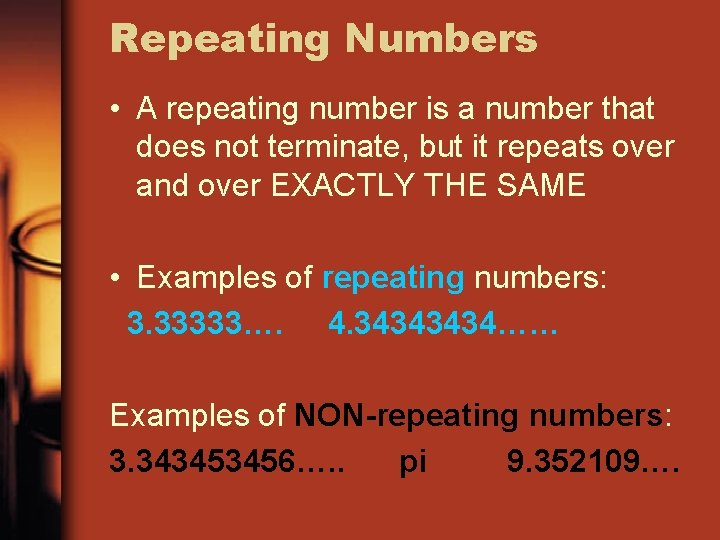Repeating Numbers • A repeating number is a number that does not terminate, but it repeats over and over EXACTLY THE SAME • Examples of repeating numbers: 3. 33333…. 4. 3434…… Examples of NON-repeating numbers: 3. 343453456…. . pi 9. 352109….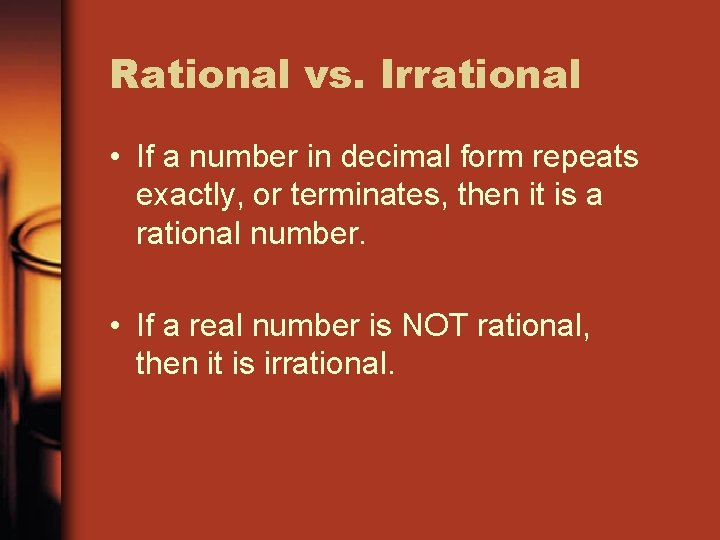Rational vs. Irrational • If a number in decimal form repeats exactly, or terminates, then it is a rational number. • If a real number is NOT rational, then it is irrational.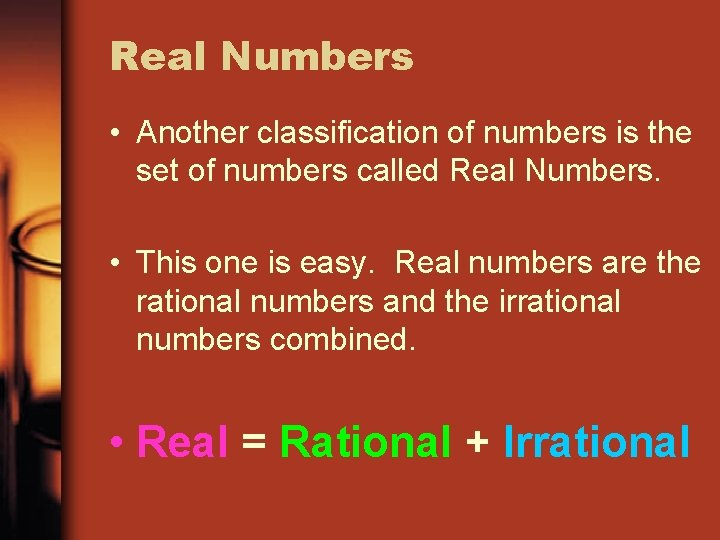Real Numbers • Another classification of numbers is the set of numbers called Real Numbers. • This one is easy. Real numbers are the rational numbers and the irrational numbers combined. • Real = Rational + Irrational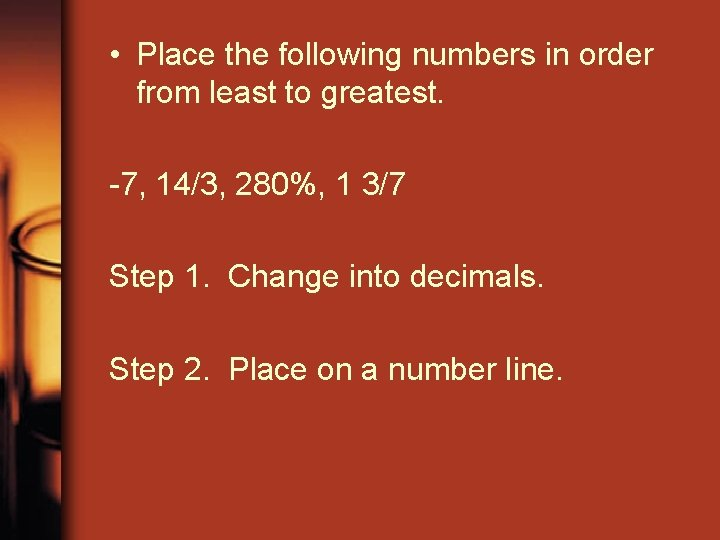• Place the following numbers in order from least to greatest. -7, 14/3, 280%, 1 3/7 Step 1. Change into decimals. Step 2. Place on a number line.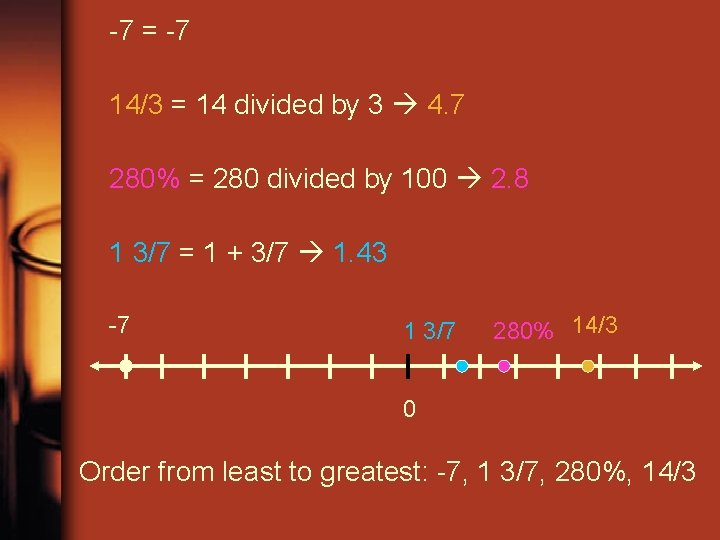-7 = -7 14/3 = 14 divided by 3 4. 7 280% = 280 divided by 100 2. 8 1 3/7 = 1 + 3/7 1. 43 -7 1 3/7 280% 14/3 0 Order from least to greatest: -7, 1 3/7, 280%, 14/3